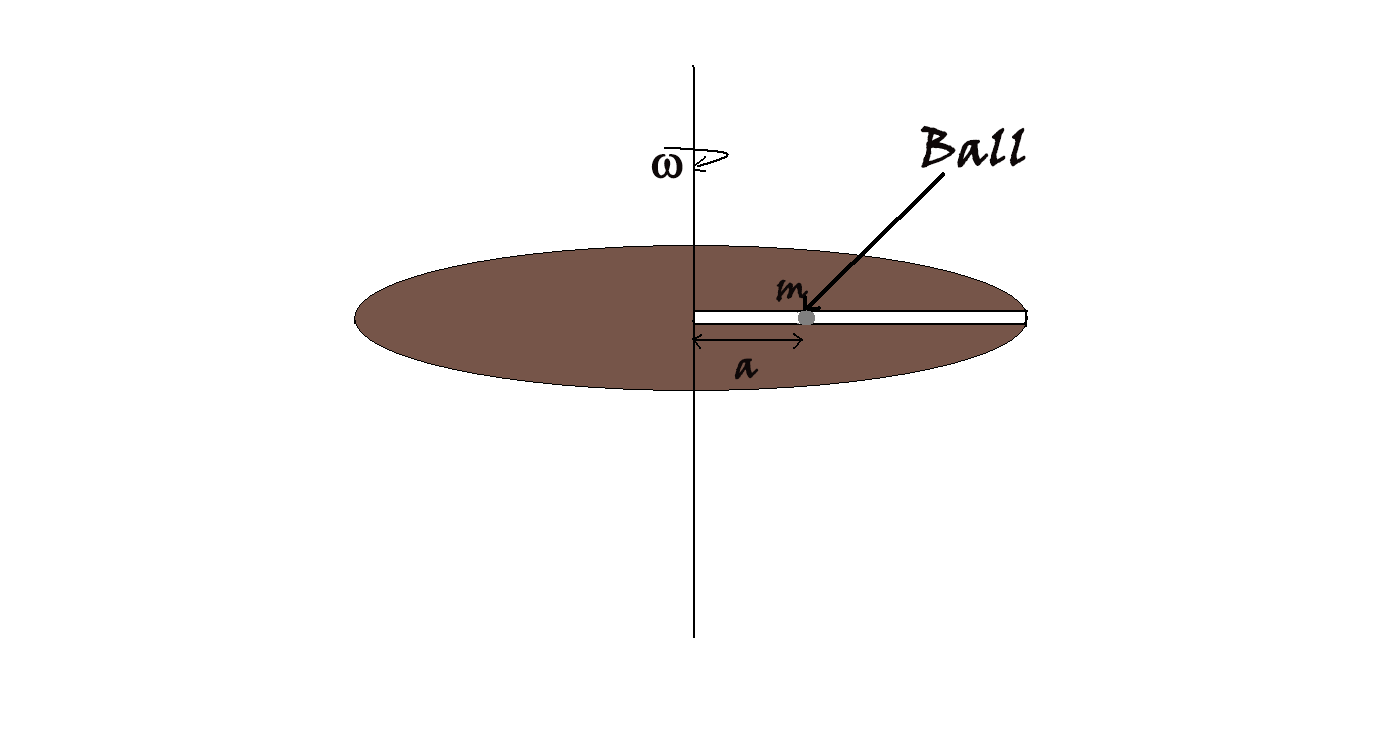Rotating turntable with a ballThe turntable shown in figure is rotated with constant $\omega = 2$ rad/sec. A ball of mass 3 kg is gently placed in a frictionless tunnel at a distance $3\sqrt3$ m from the center. Find the radial velocity of ball (in m/s) when it reaches at the end of the tunnel provided, the length of the tunnel is 14 m.

×